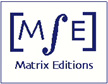Matrix Editions
serious mathematics,
written with the reader in mind

### Functional Analysis Volume 1: A Gentle Introduction by Dzung Minh Ha

ISBN 9780971576612
640 pages, published 2006. Hardcover, smythe-sewn binding

The hardcover version of this book is currently out of print. It can be ordered as a pdf ebook. Please read this before ordering.

pdf ebook, \$71.00

### Chapter 1: Metric and topological spaces

1.1 Metrics and metric spaces
1.2 Open and closed sets
1.3 Topological spaces
1.4 Continuous functions
1.5 Open sets and continuity
1.6 Some important topological concepts
1.7 Convergence of sequences in metric spaces
1.8 Completeness
1.9 Density, separability, and approximation
1.10 Metric space completions
1.11 Compactness
1.12 The Banach fixed point theorem
1.13 Baire's category theorem

### Chapter 2: Normed spaces 116

2.1 Linear operators on function spaces
2.2 Hamel bases
2.3 Norms and normed spaces
2.4 Topological concepts in normed spaces
2.5 Topological vector spaces
2.6 Kolmogorov's theorem
2.7 Banach spaces
2.8 Infinite series in normed spaces
2.9 Schauder bases
2.10 Linear functionals and hyperplanes
2.11 Constructing new normed spaces

### Chapter 3: Operators on normed spaces

3.1 Continuous linear maps
3.2 Integral operators
3.3 Linear homeomorphisms
3.4 Three important theorems
3.5 The normed space B(X,Y)
3.6 Complementary subspaces and projections
3.7 Riesz's lemma
3.8 The spectrum of a bounded linear operator
3.9 Continuous linear functionals and dual spaces

### Chapter 4: Inner product spaces

4.1 Definitions and examples
4.2 Orthogonality
4.3 Unitary isomorphisms
4.4 Inner product spaces: three problems
4.5 Three characterizations for Hilbert spaces
4.6 Hilbert bases

### Chapter 5: The Banach space C(X)

5.1 The Arzela-Ascoli theorem
5.2 Korovkin's theorem and the Weierstrass approximation theorem
5.3 Sub-algebras
5.4 The Stone-Weierstrass theorem

6.1 The Baire-Osgood theorem
6.2 Gram determinants and Muntz's theorem
6.3 Differential equations

### Appendix A: Set theory and functions

A.1 Sets
A.2 Relations
A.3 Zorn's lemma and the axiom of choice
A.4 Functions
A.5 Cardinality
A.6 The axiom of completeness on R

### Appendix B: Mostly linear algebra (a brief review)

B.1 Polynomials and sequences
B.2 Vector spaces
B.3 Linear independence and span
B.4 Bases and dimension
B.5 Linear transformations
B.6 Partial derivatives and the mean value theorem
B.7 Riemann integrals